#### You may also like### Consecutive Numbers

An investigation involving adding and subtracting sets of consecutive numbers. Lots to find out, lots to explore.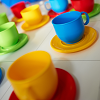### Tea Cups

Place the 16 different combinations of cup/saucer in this 4 by 4 arrangement so that no row or column contains more than one cup or saucer of the same colour.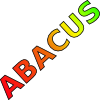### Counting on Letters

The letters of the word ABACUS have been arranged in the shape of a triangle. How many different ways can you find to read the word ABACUS from this triangular pattern?

##### Age 11 to 14Challenge Level

Take an addition table from $1$ to $10$ (or any other that you like better!):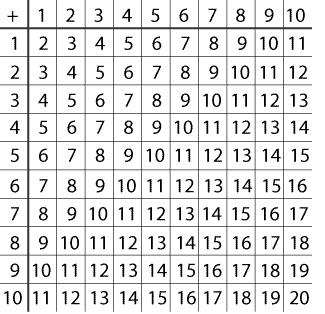Now take a $3\times 3$ square of numbers on it, such as this red one: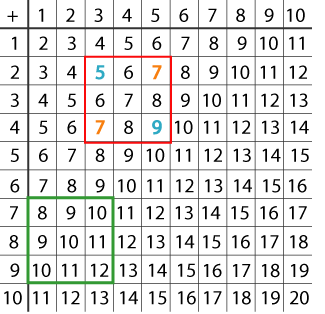Now multiply the two diagonally opposite corner numbers together: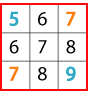And then find the difference between the two answers:
$5 \times 9 = 45$
$7 \times 7 = 49$
$49 - 45 = 4$
Now try the numbers in the green square: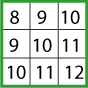Investigate other $3\times 3$ squares.
Now try with $2\times 2$ squares and $4\times 4$ squares.